Reach Us+44-1522-440391
Investigation on the Exergy Performance of a Central Receiver Power Plant | OMICS International
Journal of Fundamentals of Renewable Energy and Applications
All submissions of the EM system will be redirected to Online Manuscript Submission System. Authors are requested to submit articles directly to Online Manuscript Submission System of respective journal.

# Investigation on the Exergy Performance of a Central Receiver Power Plant

DI Ramadan Ali Abdiwe and Markus Haider*

Vienna University of Technology, Institute for Exergy Systems and Thermodynamics, Austria

Corresponding Author:
Markus Haider
Professor, Vienna University of Technology
Institute for Exergy Systems and Thermodynamics
Getreidemarkt 9, 1060 Wien, Austria
Tel: + 43 (1) 58801 – 302301
E-mail: [email protected]

Received date: May 25, 2015; Accepted date: June 22, 2015; Published date: June 30, 2015

Citation: Abdiwe DRA, Haider M (2015) Investigation on the Exergy Performance of a Central Receiver Power Plant. J Fundam Renewable Energy Appl 5:168. doi:10.4172/2090-4541.1000168

Copyright: © 2015 Abdiwe DRA, et al. This is an open-access article distributed under the terms of the Creative Commons Attribution License, which permits unrestricted use, distribution, and reproduction in any medium, provided the original author and source are credited.

Visit for more related articles at Journal of Fundamentals of Renewable Energy and Applications

#### Abstract

The present paper describes the exergy analysis of a Central Receiver System (CRS) power plant. The plant consists of a thousand heliostats with an area of 130 m² each, an external receiver with an area of 59 m² and a height of 70 m, a steam generator, two steam turbines with a reheater in between, two feed water heaters and a condenser. EBSILON®Professional software was used to obtain the exergy efficiency and the irreversibility in each component of the power plant to pinpoint the causes and locations of the thermodynamic imperfection. The model analyzed and tested the effect of two design parameters including the Direct Normal Irradiation (DNI) and the outlet temperature of the Heat Transfer Fluid (HTF) on the exergy performance. The obtained results show at a constant DNI the maximum exergy loss occurs at the Receiver followed by the heliostat field and the power cycle has the lowest exergy loss. The increase of the DNI affects negatively the exergy efficiency of the overall system. The variation of the outlet temperature of the HTF has an impact of the exergy performance of the receiver subsystem as well as the overall system; the increase of the outlet temperature from 450˚C to 600˚C leads to an increase the exergy efficiency of the receiver to about 5% and an increase the exergy efficiency of the overall system to about 1%.

#### Nomenclature

ηII:          Exergy efficiency(%),

Wnet         Net output of the overall system (W)

Wturbine         Output of both turbines (W)

Wpump         Work for both pumps (W)

y & z Mass fractions

mst         Steam mass flow rate (kg/s)

mms         Molten salt mass flow rate (kg/s)

To         Atmospheric temperature (˚C)

Twi         Inlet water at the condenser (˚C)

Itotal         Overall system irreversibility (W)

Iheliostat         Heliostat field irreversibility (W)

Ipcycle         Power cycle irreversibility (W)

Q*total         Total isolation (W)

Q*inc         Incident isolation (W)

Q(inc,abs)         Heat absorbed by the receiver (W)

Q(inc,loss)         Heat lost at the receiver (W)

ψ*total         Exergy input into the heliostat field (W)

ψ*inc         Exergy delivered to the receiver (W)

ψ(inc,abs)         Exergy absorbed by the receiver (W)

HP-T High pressure turbine

LP-T Low pressure turbine

OFWH Open feed water heater

CFWH Close feed water heater

CO Condenser

EV Evaporator

S-H Solar Heater

#### Introduction

Solar exergy is an important alternative exergy source used in many applications, especially in solar power systems which utilize the heat generated by collectors concentrating and absorbing the sun’s exergy to drive heat engines/generators and produce electric power . Most known types of the solar-thermal systems to produce electricity are trough/steam turbine, tower/steam turbine, and dish/heat engine systems. Out of all these technologies, tower/steam turbine looks like to be the best choice for high power production as it has the largest operating temperature range . Tower/steam turbine or what so called Central Receiver System (CRS) is composed of the following main components: the heliostats, the receiver and the power block. The thermal storage and balance of plant components allow high temperatures which lead to high efficiency of the power conversion system . However, the power generation efficiency of the CRS systems are found to be low which directly increase the capital cost of the electricity generation. To investigate the cause of the low generation efficiency in the power generation system an exergy analysis is required. The exergy analysis has proven to be a powerful tool in thermodynamic analyses of the system . Therefore, a theoretical investigation based on the second law efficiency has been conducted for a CRS power plant.

The Exergy is the maximum useful work that can be obtained from a system at a given state in a given environment; in other words, the most work you can get out of a system. In the last several decades exergy analysis has begun to be used for system optimization by analyzing the exergy destroyed in each component in a process. With the exergy analysis we can see where we should be focusing our efforts to improve system efficiency. The exergy analysis method is a useful tool for promoting the goal of more efficient exergy-resource use, as it enables the locations, types and true magnitudes of wastes and losses .

However, few papers have appeared on the exergy analysis and performance assessment of the solar thermal power plant. Bejan  showed that the amount of useful exergy (exergy) delivered by solar collector systems is affected by heat transfer irreversibility occurring between the sun and the collector, between the collector and the ambient air, and inside the collector. Singh  stated that the collector-receiver assembly is the part where the losses are maximum and the maximum exergy loss occurs in the solar collector field. Gupta and Kaushik  made an exergy analysis for the different components of a proposed conceptual direct steam generation (DSG) solar–thermal power plant and found that the maximum exergy loss is in the solar collector field while in other plant components is small. Chao  evaluated the exergy losses in each component and in a power tower solar plant and the results showed that the maximum exergy loss occurs in the receiver system.

The objective of this paper is to evaluate the exergy efficiencies and the irreversibilities of all components of a CRS power plant with an external central receiver and a supercritical Rankine cycle and pinpoint the causes and locations of the thermodynamic imperfection and the magnitude of the process irreversibilities in the system. Two parameters have been varied to see their effect on the exergy efficiency of the receiver and the overall system.

#### System Configuration

The schematic of the solar tower power plant is shown in Figure 1. The system consists of a heliostat field system, an external central receiver, a steam generator, two steam turbines with a reheat in between, a wet condenser, open and close feed water heaters and two pumps.

The target is to do an exergy analysis for each component of the power plant including the power cycle, the receiver and the heliostat to have more profound understanding of the performance of the CRS power plant. Also to compare all components and see which one has the biggest fractional exergy loss which will provide the guides of improving the performance and optimizing the operation.

Assumptions

The following assumptions are made in the analysis:

a. The system runs of steady state with a constant solar insulation

b. Capacity of the power plant is 30MW

c. The CRS power plant is using molten salt as HTF

d. The temperature of molten salt entering the receiver 290˚C and leaving it 565˚C

e. Kinetic and potential exergy are ignored

f. The optical efficiency of the receiver is assumed to be 93%

g. Conductive heat loss in the receiver is ignored

The Exergy Analysis

The Second Law Efficiency or what so called the exergy efficiency of the whole system can be defined as the ratio of net electricity output from the whole system to the exergy input associated with the solar irradiation on the heliostat surface . The exergy input into the heliostat field (ψ*total) is basically the electricity output (Wnet) plus the irreversibility (I_total ) which is also called the exergy destroyed. The exergy destroyed represents exergy that could have been converted into work but was instead wasted. Therefore, the exergy efficiency of the overall system is given by: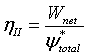(1)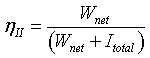(2)

Where the net output work (electricity) from the whole system is calculated as: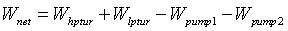(3)

The total irreversibility (Itotal) of the whole system is the summation of the irreversibility of the subsystems: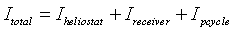(4)

Power cycle

To improve the exergy efficiency of the power cycle a regenerative Rankine cycle is used as the case of most existed solar tower power plants. The power cycle of our system as shown in Figure 1 consists of high and low-pressure turbine stages, a condenser, two pumps and feed water heater. Since a molten salt is being used as Heat Transfer Fluid, it is necessary to use an open and a closed feed water heaters to prevent the solidification of the molten salt in the steam generation subsystem.

The exergy efficiency of the power cycle subsystem can be calculated as: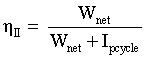(5)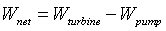(6)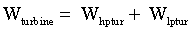(7)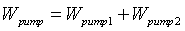(8)

In order to calculate the power cycle output (Wnet), it is necessary to apply an exergy balance in the regenerative Rankine cycle. As a result the power delivered by the turbines and consumed by the pumps can be evaluated as following: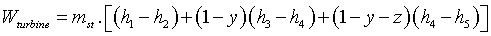(9)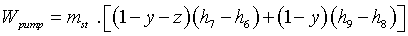(10)

y & z are the mass fractions and can be calculated by applying an exergy balance on the open and the closed feed water heaters. Therefore, the values of the mass fractions are: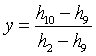(11)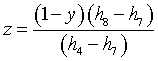(12)

The irreversibility of the power cycle (Ipcycle) is the summation of the irreversibility of each component of the power cycle subsystem.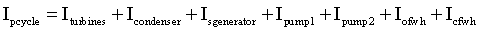(13)

The entropy generation in a system is the cause of the exergy destruction. The destruction of useful work because of the entropy generated knows also as irreversibility. The exergy destruction is a proportional of the entropy generated. The general equation for the exergy destruction can be expressed as: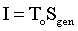(14)

Sgen is the entropy generated, for a steady state control volume, this leads us to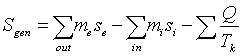(15)

where

mi and me are the mass flow rate in and out respectively.

si and se are the entropy in and out respectively.

Q is the heat loss to the surrounding.

Tk is the temperature of the heat source.

As a result, the irreversibility of each component of the power cycle can be defined as: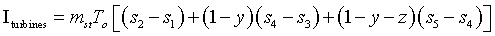(16)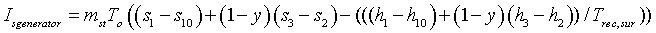(17)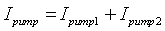(18)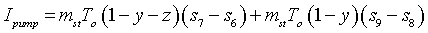(19)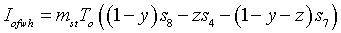(20)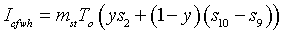(21)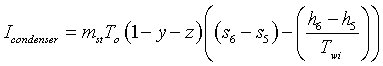(22)

In the solar receiver, the heat flux arriving at the aperture area is transferred into a heat flow directed to the HTF. The heat is used to raise the temperature of the HTF. On the way from the aperture to the fluid, some optical and thermal losses occur. Therefore, the incident isolation can be obtained by: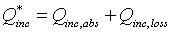(23)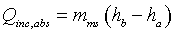(24)

ha and hb are the inlet and exist enthalpy of the molten slat at the receiver respectively.

Regarding the thermal losses, only the radiation and convective losses have been considered and the others are partially small and to be ignored.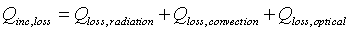(25)

The radiation and convection heat loss is obtained from a CFD model for the same receiver geometry and same boundary conditions done by the author . In the CFD model a Simulation tool ANSYS® FLUENT® was used to determine the thermal heat loss in both cavity and external (CRS) at wind speed varies from (2) to (10) m/s. Table 1 shows the values of the thermal heat loss at the receiver.

 DNI [W/m2] Wind speed [m/s] Radiation loss [kW] Convection loss [kW] 800 4 774 251

Table 1: Thermal loss at the receiver .

The optical efficiency is assumed to be 93%. Therefore, the Q(loss,optical) is equal to 0,07Q*inc

The exergy delivered to the receiver can be calculated by :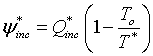(26)

T* is the apparent sun temperature as an exergy source and will considered 4500 K.

Similarly the exergy absorbed in the receiver can be given by: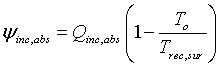(27)

The exergy efficiency of the receiver is defined as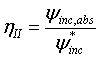(28)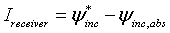(29)

Heliostat field system

The incoming solar irradiance onto the receiver aperture area is concentrated by a large number of individually tracked heliostats. The flux density distribution on a defined aperture surface is the output of the heliostat field. This aperture surface can be used as an interface between the optical concentrator and the solar receiver.

The total isolation (Q*total) is a proportional to the heliostat field aperture area and can be given by :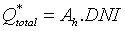(30)

where

Ah is the heliostat field aperture area.

DNI is the direct normal irradiation.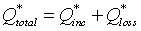(31)

The total exergy (ψ*total) associated with the solar irradiation on the heliostat mirror surface (Q*total) can be expressed as :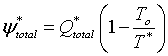(32)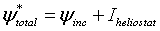(33)

The exergy efficiency of the heliostat will be as: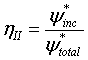(34)

The irreversibility in the heliostat is: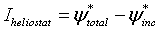(35)

#### Fractional Exergy Loss

The ratio of the irreversibility of each component to the irreversibility of the whole system is the fractional exergy loss for that component. That illustrates the loss of useful exergy in each component which helps us to decide where we should be focusing our efforts to improve system efficiency.

The fractional exergy loss of each component can be calculated as: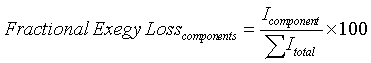(36)

#### Results

The exergy analysis has been carried out for a CRS power plant with Rankine cycle using one open and closed feed water heater. At a constant DNI the exergy efficiency differs from a component to another. The highest exergy efficiency is associated with the power cycle subsystem meanwhile the receiver subsystem has the lowest exergy analysis. It can be seen from the graph in the Figure 2 that at fixed DNI 800 W/m2 and also fixed Outlet temperature of the HTF (molten salt) 565˚C, the exergy efficiencies are 80%, 74% and 58% for the power cycle, the heliostat and the receiver respectively.

Figure 3 illustrates the effect of the DNI on the fractional exergy loss. The fractional loss at each component of the power cycle subsystem decreases slightly with the increase of the DNI. However, at the receiver and the heliostat field subsystems the case is opposite. The fractional loss at the receiver and the heliostat field increases with the increase the DNI and as a result the exergy efficiency in both subsystems decreases.

Figure 3: The effect of the direct normal irradiation on the fractional exergy loss of all components.

The total isolation (Q*total) at the heliostat field and the exergy (ψ*total) associated with it are both a proportional of the DNI as illustrated in equations (30) and (32). However, the second law efficiency of the heliostats (ηII,heliosat) is inversely proportional of the DNI and it gets lower because the irreversibility of the heliostat gets higher as shown in equation (34). Similarly when the DNI increase the exergy delivered to the receiver (ψ*inc) increase and also the irreversibility at the receiver increase (Ireceiver) and this leads to the decrease in the exergy efficiency of the receiver (ηII,receiver) as described in the equation (28).

Figure 4 shows the effect of the DNI on the exergy efficiency of the whole system. Since the irreversibility at the receiver and the heliostat field increase with the increase of the DNI, the total irreversibility (Itotal) increases and as a result the exergy efficiency of the whole system decreases.

The variation in the exergy efficiency of both the receiver and the overall system with the outlet temperature of the molten salt is shown in the Figure 5. It can be seen that the exergy efficiency at the receiver and the overall system both increase to a certain degree with the increase of the outlet temperature. The outlet temperature has more effect on the exergy efficiency of the receiver subsystem with comparison to the overall system. The increase of the outlet temperature of the molten salt from 450˚C to 600˚C will lead to an increase in exergy efficiency at the receiver to about 5% while the increase of the exergy efficiency at the overall system is only in the range of 1%.

Figure 5: The effect of outlet temperature of the HTF (molten salt) on the exergy efficiency of the receiver and the whole system.

The fractional exergy loss at the receiver and the heliostat subsystems varies with different values of the outlet temperature as shown in Figure 6. Regarding the receiver subsystem the fractional exergy loss decreases with the increase of the outlet temperature. However, the fractional exergy loss at the heliostat field subsystem increases and this lowers the exergy efficiency. As the outlet temperature of the molten salt gets higher the flow rate should be lowered in order to heat the molten salt to the required temperature and therefore, the absorbed heat at the receiver by the molten salt (Qinc, abs) is diminishing. As shown in equation (27) the exergy delivered to the receiver (ψinc,abs) is proportional of the absorbed heat by the receiver (Qinc, abs) Therefore, the exergy delivered to the receiver (ψinc,abs) also reduced and as a result the exergy efficiency of the receiver decreases as illustrated in equation (28). It can be also seen from the Figure 6 that the variation of the outlet temperature of the molten salt has no effect on the exergy efficiency of the power cycle subsystem components.

Figure 6: The effect of the outlet temperature of the HTF (molten salt) on the fractional exergy loss of all the components in the system.

#### Conclusions

In this paper, a theoretical investigation based on the second law efficiency has been conducted for a CRS power plant with an external central receiver and a supercritical Rankine cycle to pinpoint the causes and locations of the thermodynamic imperfection and the magnitude of the process irreversibilities in the system. The following conclusions can be drawn from study:

The receiver subsystem has the highest fractional exergy loss and as a result it has the lowest exergy efficiency (58%) followed by the heliostat field subsystem (74%). While the power cycle subsystem having the lower fractional exergy loss and as result the highest exergy efficiency (80%).

The variation of the DNI affects the fractional exergy loss in all components. The increase of the DNI leads to a decrease of the fractional exergy loss in the power cycle subsystem components and an increase of the fractional exergy loss in the receiver and heliostat field subsystems. As result the increase of the DNI affects negatively the exergy efficiency of the overall system.

The variation of the outlet temperature of the HTF (molten salt) has no significant impact on the exergy efficiency of the receiver subsystem as well as the overall system. It is found that by increasing the outlet temperature of the molten salt from 450˚C to 600˚C will increase the exergy efficiency of the receiver subsystem to almost 5% and almost 1% to the exergy efficiency of the overall system.

#### Acknowledgements

The author is thankful for the institute for Exergy Systems and Thermodynamics at Vienna Technical University (TU). I am also grateful to Professor Haider for the help provided in guiding the study.

#### References

Select your language of interest to view the total content in your interested language

### Article Usage

• Total views: 12606
• [From(publication date):
July-2015 - Jul 21, 2019]
• Breakdown by view type
• HTML page views : 8693Can't read the image? click here to refresh